# Gaussian curvature

of a surface

The product of the principal curvatures (cf. Principal curvature) of a regular surface at a given point.

If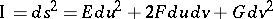is the first fundamental form of the surface and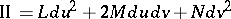is the second fundamental form of the surface, then the Gaussian curvature can be computed by the formula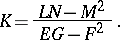The Gaussian curvature is identical with the Jacobi determinant of the spherical map: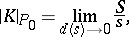where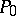is a point on the surface,is the area of a domainwhich contains,is the area of the spherical image of, andis the diameter of the domain. The Gaussian curvature is positive at an elliptic point, negative at a hyperbolic point, and is zero at a parabolic point or a flat point. It may be expressed in terms of the coefficients of the first fundamental form and their derivatives alone (the Gauss theorem), viz.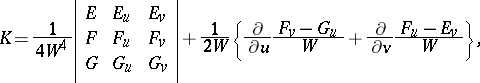whereSince the Gaussian curvature depends on the metric only, i.e. on the coefficients of the first fundamental form, the Gaussian curvature is invariant under isometric deformation (cf. Deformation, isometric). The Gaussian curvature plays a special role in the theory of surfaces, and many formulas are available for its computation, .

The concept was introduced by C.F. Gauss , and was named after him.